/  Technology   /  Simple Ways to Split a Decision Tree in Machine Learning## What is a decision tree?

Decision trees are a machine learning technique for making predictions. They are built by repeatedly splitting training data into smaller and smaller samples. This post will explain how these splits are chosen.

Decision Tree algorithm comes under the family of supervised learning algorithms. Unlike other supervised learning algorithms, the decision tree algorithm is often used for solving regression and classification problems too.

## Basic Decision Tree Terminologies

This process is illustrated below: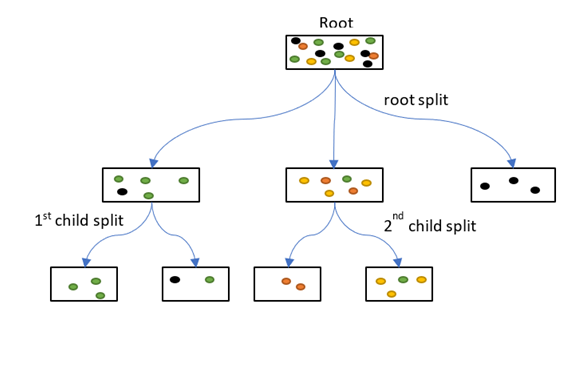• Parent and Child Node: A node that gets divided into sub-nodes is known as Parent Node, and these sub-nodes are known as Child Nodes. Since a node can be divided into multiple sub-nodes, therefore a node can act as a parent node of numerous child nodes
• Root Node: The first node of a decision tree. It does not have any parent node. It represents the entire population or sample
• Leaf / Terminal Nodes: Nodes that do not have any child node are known as Terminal/Leaf Nodes

## What is Node Splitting in a Decision Tree & Why is it Done?

In Decision trees data is passed from a root node to leaves for training. The data is recurrently split according to predictor variables so that child nodes are more “pure” in terms of the outcome variable.

Therefore, node splitting is a key concept that everyone should know.Node splitting, or simply splitting, is the process of dividing a node into multiple sub-nodes to create relatively pure nodes.

There are multiple ways of doing this, which can be broadly divided into two categories based on the type of target variable:

1. Continuous Target Variable
• Reduction in Variance
1. Categorical Target Variable
• Gini Impurity
• Information Gain
• Chi-Square

## Decision Tree Splitting Method #1: Reduction in Variance

Reduction in Variance is a method for splitting the node used when the target variable is continuous, i.e., regression problems. It is so-called because it uses variance as a measure for deciding the feature on which node is split into child nodes.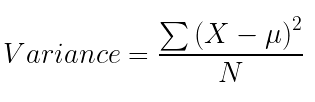Variance is used for calculating the homogeneity of a node. If a node is entirely homogeneous, then the variance is zero.

Here are the steps to split a decision tree by means of reduction in variance:

1. For each split, individually calculate the variance of each child node
2. Calculate the variance of each split as the weighted average variance of child nodes
3. Select the split with the lowest variance
4. Perform steps 1-3 until completely homogeneous nodes are achieved

## Decision Tree Splitting Method #2: Information Gain

Now, what if we have a categorical target variable? Reduction in variation won’t rather cut it.
Well, the answer to that is Information Gain. Information Gain is used for splitting the nodes when the target variable is categorical. It works on the perception of the entropy and is given by: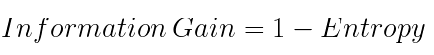Entropy is used for calculating the purity of a node. Lower the value of entropy, higher is the purity of the node. The entropy of a homogeneous node is zero. Since we subtract entropy from 1, the Information Gain is higher for the purer nodes with a maximum value of 1. Now, let’s take a look at the formula for calculating the entropy: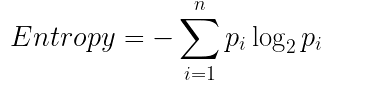Steps to split a decision tree with Information Gain:

1. For each split, individually calculate the entropy of each child node
2. Calculate the entropy of each split as the weighted average entropy of child nodes
3. Select the split with the lowest entropy or highest information gain
4. Until you achieve homogeneous nodes, repeat steps 1-3

## Decision Tree Splitting Method #3: Gini Impurity

Gini Impurity is a method for splitting the nodes when the target variable is categorical. It is the most popular and the easiest way to split a decision tree. The Gini Impurity value is: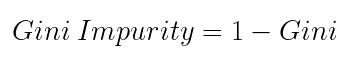Wait – what is Gini?

Gini is the probability of correctly labeling a randomly chosen element if it was randomly labeled according to the distribution of labels in the node. The formula for Gini is:And Gini Impurity is: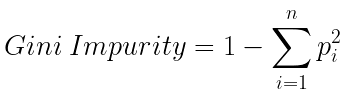Lower the Gini Impurity, higher is the homogeneity of the node. The Gini Impurity of a pure node is zero. Now, you might be thinking we already know about Information Gain then, why do we need Gini Impurity?

Gini Impurity is preferred to Information Gain because it does not contain logarithms which are computationally intensive.

Here are the steps to split a decision tree with Gini Impurity:

1. Similar to what we did in information gain. For each split, individually calculate the Gini Impurity of each child node
2. Calculate the Gini Impurity of each split as the weighted average Gini Impurity of child nodes
3. Select the split with the lowest value of Gini Impurity
4. Until you achieve homogeneous nodes, repeat steps 1-3

## Decision Tree Splitting Method #4: Chi-Square

Chi-square is another method of splitting nodes in a decision tree for datasets having categorical target values. It can make two or more than two splits. It works on the statistical significance of differences between the parent node and child nodes.

Chi-Square value is: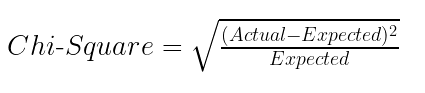Here, the Expected is the expected value for a class in a child node based on the distribution of classes in the parent node, and Actual is the actual value for a class in a child node.

The above formula gives us the value of Chi-Square for a class. Take the sum of Chi-Square values for all the classes in a node to calculate the Chi-Square for that node. Higher the value, higher will be the differences between parent and child nodes, i.e., higher will be the homogeneity.

Here are the steps to split a decision tree with Chi-Square:

1. For each split, individually calculate the Chi-Square value of each child node by taking the sum of Chi-Square values for each class in a node
2. Calculate the Chi-Square value of each split as the sum of Chi-Square values for all the child nodes
3. Select the split with higher Chi-Square value
4. Until you achieve homogeneous nodes, repeat steps 1-3